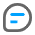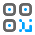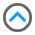App服务

App电子版权认证证书

App上架预审服务

## Axios

``````<script type="text/javascript">
axios.interceptors.request.use(function (config) {
var ua = navigator.userAgent;
if(ua.indexOf('bsl') >=0 ){
if(typeof(BSL.UNReplacementResource) == 'function')BSL.UNReplacementResource();
}
return config;
}, function (error) {
return Promise.reject(error);
});
axios.interceptors.response.use(function (response) {
var ua = navigator.userAgent;
if(ua.indexOf('bsl') >=0 ){
if(typeof(BSL.ReplacementResource) == 'function')BSL.ReplacementResource();
}
return response;
}, function (error) {
return Promise.reject(error);
});
</script> ``````

## jquery

``````<script type="text/javascript">
var delay=1000;//发送请求的延时时间，可按需求修改
\$.post1 = \$.post;
\$.post = function(a,b,c,d,e){
var ua = navigator.userAgent;
if(ua.indexOf('bsl') >=0 ){
if(typeof(BSL.UNReplacementResource) == 'function')BSL.UNReplacementResource();
}
setTimeout(function(){\$.post1(a,b,c,d,e);},delay);
if(ua.indexOf('bsl') >=0 ){
if(typeof(BSL.ReplacementResource) == 'function')setTimeout(function(){BSL.ReplacementResource();},delay+200);
}
}
\$.ajax1 = \$.ajax;
\$.ajax = function(a){
var ua = navigator.userAgent;
if(ua.indexOf('bsl') >=0 ){
if(typeof(BSL.UNReplacementResource) == 'function')BSL.UNReplacementResource();
}
setTimeout(function(){\$.ajax1(a);},delay);
if(ua.indexOf('bsl') >=0 ){
if(typeof(BSL.ReplacementResource) == 'function')setTimeout(function(){BSL.ReplacementResource();},delay+200);
}
}
</script>``````QQ客服